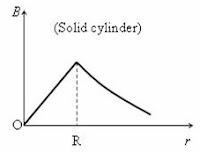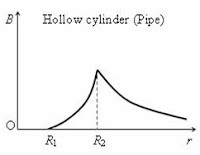## Pages

`“Life is like riding a bicycle.  To keep your balance you must keep moving.”–Albert Einstein`

## Friday, June 10, 2011

### AP Physics C – Applications of Ampere’s Circuital Law – Magnetic Field due to Straight Infinitely Long Thick Current Carrying Cylinders and Pipes

In the post dated 1st February 2008 the equations to be noted in connection with magnetic fields were given. [To access that post click here]. Ampere’s circuital law was briefly mentioned there.

Ampere’s circuital law states that the line integral of magnetic flux density B over any closed curve is equal to µ0 times the total current I passing through the surface enclosed by the closed curve. This is stated mathematically as

B. dℓ = µ0I (The integration is over the closed path)

[Ampere’s circuital law as modified by Maxwell to accommodate the displacement current flowing through dielectrics and free space is

B. dℓ = µ0 [I+ ε0 (dφE/dt)], where ε0 (dφE/dt) is the displacement current resulting from the rate of change of electric flux φE. ε0 is the permittivity of free space].

In this post I want to make you realize the importance of Ampere’s circuital law as a simple tool for evaluating the magnetic field in situations exhibiting symmetry in such a manner that the magnitude of the magnetic field is the same along the path chosen for the line integral.

You might have used Ampere’s circuital law to derive the expression, B = µ0µrnI for the magnitude of the magnetic flux density inside very long solenoids and toroids. [Here µ0 is the permeability of free space, µr is the relative permeability of the medium filling the interior of the solenoid (or toroid), n is the number of turns per metre and I is the current].

You might have certainly used Ampere’s circuital law to derive the expression, B = μ0I/2πr for the magnitude of the magnetic flux density at a point P distant ‘r’ from an infinitely long straight conductor carrying a steady current I. The above expression shows that the magnetic field is inversely proportional to the distance of the point P from the conductor. Normally you won’t worry about the variation of the magnetic field inside the conductor since the conductor is assumed to be thin. Now, suppose the conductor is thick. Now you have enough space to move about inside the body of the conductor to explore the variation of the magnetic field!

Let us use Ampere’s circuital law for finding the magnetic field due to a thick cylindrical conductor of radius R. A cross section of the thick conductor is shown in the following figure.

The conductor is straight and infinitely long, with its length normal to the plane of the figure. The current I is supposed to flow into the plane of the figure, away from the reader.

Consider a point P at distance r from the centre of the conductor such that r < R so that the point P is within the conductor. This is a situation exhibiting symmetry and the magnitude B of the magnetic flux density at all points at the same distance r from the centre must be the same. Applying Ampere’s circuital law, we equate the line integral of the magnetic field vector B over a circular path (red dotted circle) of radius r to the product of µ0 and the appropriate current:

B. dℓ = µ0I1 ………….(i)

where I1 is the current passing (piercing) through the surface enclosed by the circular path of radius r. Note that I1 is the current carried by the cylindrical portion of the conductor having radius ‘r’so that

I1 = Ir2/πR2) = Ir2/R2

[The total current I passes through the total cross section area πR2. So the current I1 passing through the cross section area πr2 is Ir2/πR2) = Ir2/R2].

Substituting for I1 in equation (i) and remembering that the direction of the magnetic field B is along the path of integration everywhere, we have

rB = µ0Ir2/R2

Therefore, B = μ0Ir/2πR2

This shows that the magnetic field at the centre of the conductor is zero (since r = 0) and it increases linearly with the increase in distance r within the conductor. The maximum value of field is at the surface of the conductor (corresponding to r = R) and is equal to μ0I/R.

The direction of the field lines is clockwise as we have considered the current to flow into the plane of the figure, away from the reader.

The magnitude of magnetic flux density at a point such as P’ outside the conductor (at distance r > R) is given by

rB = µ0I since the entire current I passes (pierces) through the surface enclosed by the circular path of radius r. This gives

B = μ0I/r[This is the usual expression for the magnetic field due to a long straight (thin) current carrying conductor].

The variation of the magnitude of the magnetic flux density B against the distance r is shown graphically in the adjoining figure.

Now we will consider the case of a long, straight, thick cylindrical current carrying pipe of inner radius R1 and outer radius R2.

[Derivation of the magnetic field due to a current carrying pipe using Ampere’s circuital law was one of the free response questions in the AP Physics C 2011 question paper

A cross section of the pipe is shown in the adjoining figure. The current I is supposed to flow into the plane of the figure, away from the reader.Consider a point such as P at distance r such that R1 < r < R2. To find out the magnetic flux density B at the point P within the material of the pipe, we consider the line integral of B over a circular path (red dotted circle) of radius r and apply Ampere’s circuital law to obtain

B. dℓ = µ0I1 ………….(i)

where I1 is the current passing (piercing) through the surface enclosed by the circular path of radius r.

We have I1 = Iπ(r2 R12) /π(R22 R12) = I(r2 R12) /(R22 R12)

[The total current I passes through the total cross section area π(R22 R12). So the current I1 passing through the cross section area π(r2 R12) is Iπ(r2 R12) /π(R22 R12) = I(r2 R12) /(R22 R12)].

Substituting for I1 in equation (i) and remembering that the direction of the magnetic field B is along the path of integration everywhere, we have

rB = µ0I(r2 R12) /(R22 R12). This gives

B = µ0I(r2 R12) /2πr(R22 R12)

Or, B = (µ0I/r) [(r2 R12)/(R22 R12)]

This expression shows that the magnetic field is zero at r = R1 (at the inner surface of the pipe) and it increases non-linearly (unlike in the case of a solid cylindrical conductor), within the material of the pipe.

Now, what is the magnetic field inside the hollow region of the pipe? You might have definitely come across this question earlier and might have proved (by other methods) that it is zero everywhere within the hollow region. Well, Ampere’s circuital law offers an immediate proof to you:

When you consider the line integral over a concentric circular path (of the type we considered above) within the hollow region, you find that there is no current passing (piercing) through the surface enclosed by the circular path. Therefore we have

B. dℓ = µ0×0 so that B = 0

What is the magnetic field at a point outside the pipe?

We consider a point such as P’ at distance r > R2. The magnitude of magnetic flux density B at the point P’ is given by

rB = µ0I since the entire current I passes (pierces) through the surface enclosed by the circular path of radius r. This gives

B = μ0I/r[As you might have expected, this is same as the usual expression for the magnetic field due to a long straight (thin) current carrying conductor].

The nature of variation of the magnitude of the magnetic field B with the distance r is shown in the adjoining figure.

You will find an interesting question with solution in this section here.